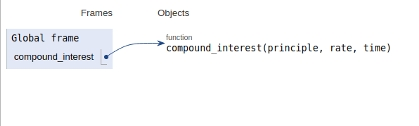# Python Program for compound interest

PythonServer Side ProgrammingProgramming

#### Beyond Basic Programming - Intermediate Python

Most Popular

36 Lectures 3 hours

#### Practical Machine Learning using Python

Best Seller

91 Lectures 23.5 hours

#### Practical Data Science using Python

22 Lectures 6 hours

In this article, we will learn about the solution and approach to solve the given problem statement.

Problem statement −We are given with three input values i.e principle, rate & time and we need to compute compound interest.

The code given below shows the process of computation of compound interest.

The formula used here is

Compound Interest = P(1 + R/100)r

Where,

P is the principal amount

R is the rate and

T is the time span

The implementation is given below

## Example

Live Demo

def compound_interest(principle, rate, time):
CI = principle * (pow((1 + rate / 100), time))
print("Compound interest : ", CI)
# main
compound_interest(10000, 7.78, 2)

## Output

Compound interest : 11616.528400000001

All variables and functions are declared in global scope as shown in the figure below.## Conclusion

In this article, we learned about the approach to compute compound interest.